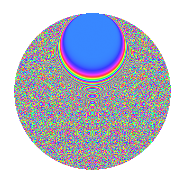# Properties

 Label 2541.2.brLevel 2541 Weight 2 Character orbit br Rep. character $$\chi_{2541}(10,\cdot)$$ Character field $$\Q(\zeta_{66})$$ Dimension 3520 Sturm bound 704

# Related objects

## Defining parameters

 Level: $$N$$ = $$2541 = 3 \cdot 7 \cdot 11^{2}$$ Weight: $$k$$ = $$2$$ Character orbit: $$[\chi]$$ = 2541.br (of order $$66$$ and degree $$20$$) Character conductor: $$\operatorname{cond}(\chi)$$ = $$847$$ Character field: $$\Q(\zeta_{66})$$ Sturm bound: $$704$$

## Dimensions

The following table gives the dimensions of various subspaces of $$M_{2}(2541, [\chi])$$.

Total New Old
Modular forms 7120 3520 3600
Cusp forms 6960 3520 3440
Eisenstein series 160 0 160

## Trace form

 $$3520q - 172q^{4} + 12q^{5} + 1760q^{9} + O(q^{10})$$ $$3520q - 172q^{4} + 12q^{5} + 1760q^{9} - 66q^{10} + 20q^{11} - 28q^{14} - 8q^{15} + 172q^{16} - 96q^{22} + 20q^{23} - 160q^{25} + 24q^{26} + 12q^{31} + 18q^{33} - 344q^{36} - 30q^{37} - 24q^{38} + 16q^{42} + 6q^{44} + 12q^{45} - 12q^{47} + 128q^{49} + 198q^{52} + 92q^{53} + 24q^{56} + 26q^{58} - 12q^{60} + 216q^{64} + 36q^{66} + 64q^{67} - 32q^{70} - 40q^{71} + 24q^{78} + 44q^{79} - 120q^{80} - 1760q^{81} + 24q^{82} - 176q^{85} - 56q^{86} + 20q^{88} + 216q^{89} + 70q^{91} + 456q^{92} - 24q^{93} + 396q^{96} + 40q^{99} + O(q^{100})$$

## Decomposition of $$S_{2}^{\mathrm{new}}(2541, [\chi])$$ into newform subspaces

The newforms in this space have not yet been added to the LMFDB.

## Decomposition of $$S_{2}^{\mathrm{old}}(2541, [\chi])$$ into lower level spaces

$$S_{2}^{\mathrm{old}}(2541, [\chi]) \cong$$ $$S_{2}^{\mathrm{new}}(847, [\chi])$$$$^{\oplus 2}$$

## Hecke Characteristic Polynomials

There are no characteristic polynomials of Hecke operators in the database